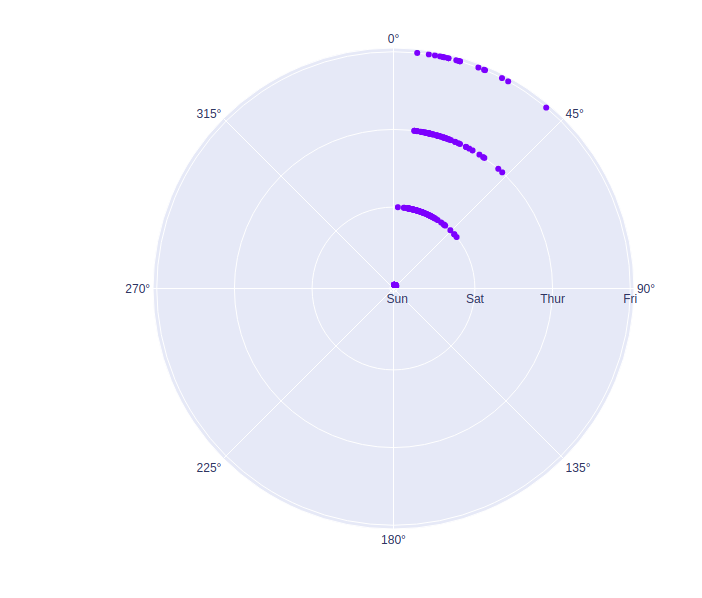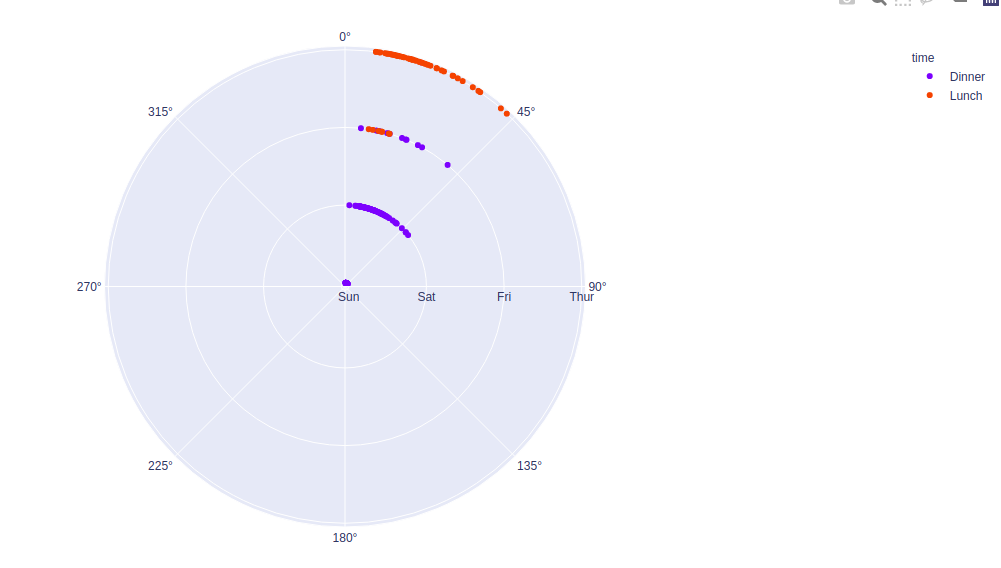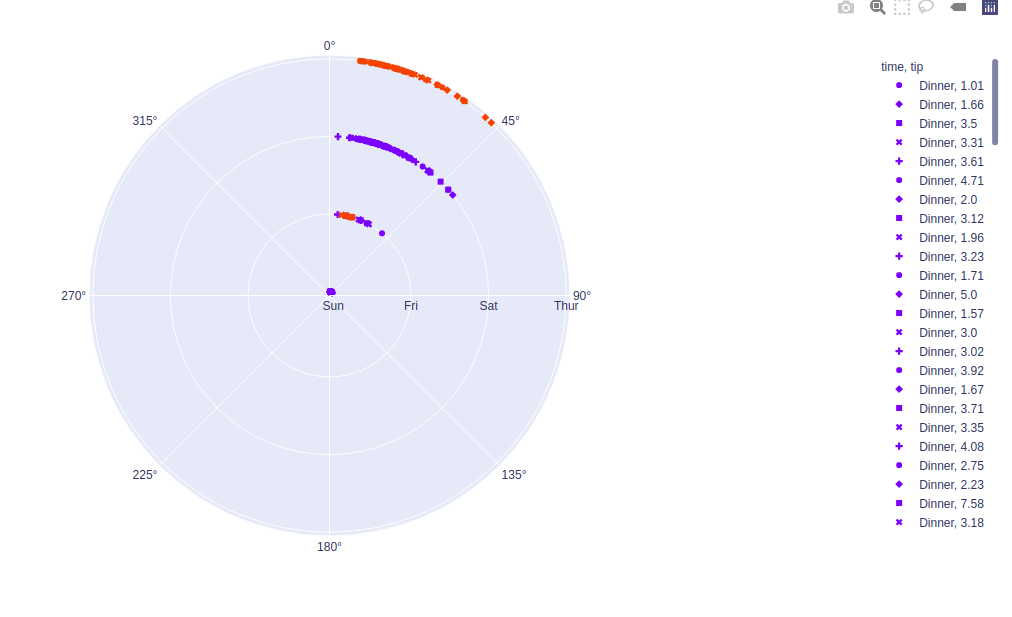# plotly.express.scatter_polar() function in Python

• Last Updated : 17 Jul, 2020

Plotly library of Python can be very useful for data visualization and understanding the data simply and easily. Plotly graph objects are a high-level interface to plotly which are easy to use.

## plotly.express.scatter_polar() function

This function is used to create a polar chart. A polar chart represents data along radial and angular axes.

Attention geek! Strengthen your foundations with the Python Programming Foundation Course and learn the basics.

To begin with, your interview preparations Enhance your Data Structures concepts with the Python DS Course. And to begin with your Machine Learning Journey, join the Machine Learning - Basic Level Course

Syntax: plotly.express.scatter_polar(data_frame=None, r=None, theta=None, color=None, symbol=None, size=None, hover_name=None, hover_data=None, title=None, template=None, width=None, height=None)

Parameters:

data_frame: DataFrame or array-like or dict needs to be passed for column names.

r, theta: This parameter is either a name of a column in data_frame, or a pandas Series or array_like object. It is used to position marks along the radial and angular axis in polar coordinates respectively.

color: This parameters assign color to marks.

symbol: This parameter is used to assign symbols to marks. It is either a name of a column in data_frame, or a pandas Series or array_like object.

size: This parameter is used to assign mark sizes. It is either a name of a column in data_frame, or a pandas Series or array_like object.

hover_name:  Values from this column or array_like appear in bold in the hover tooltip.

hover_data: This parameter is used to appear in the hover tooltip or tuples with a bool or formatting string as first element, and list-like data to appear in hover as second element Values from these columns appear as extra data in the hover tooltip.

title: The figure title

width: The figure width in pixels.

height: The figure height in pixels.

Example 1:

## Python3

 `import` `plotly.express as px`` ` `df ``=` `px.data.tips()`` ` `plot ``=` `px.scatter_polar(df, r ``=` `'day'``, ``                        ``theta ``=` `'total_bill'``)``plot.show()`

Output:Example 2: Using color argument

## Python3

 `import` `plotly.express as px`` ` `df ``=` `px.data.tips()`` ` `plot ``=` `px.scatter_polar(df, r ``=` `'day'``,``                        ``theta ``=` `'total_bill'``, ``                        ``color``=``'time'``)``plot.show()`

Output:Example 3: Using symbol argument

## Python3

 `import` `plotly.express as px`` ` `df ``=` `px.data.tips()`` ` `plot ``=` `px.scatter_polar(df, r ``=` `'day'``, ``                        ``theta ``=` `'total_bill'``,``                        ``color ``=` `'time'``,``                        ``symbol ``=` `'tip'``)``plot.show()`

Output:My Personal Notes arrow_drop_up